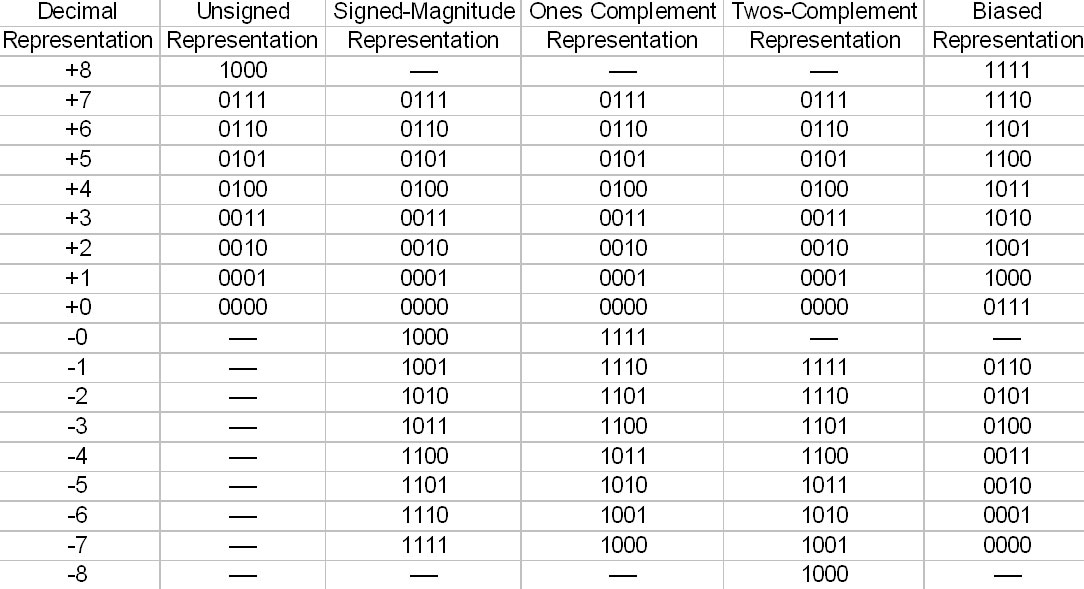Blog  /  Arduino Byte Type: A Comprehensive Guide

# Arduino Byte Type: A Comprehensive Guide

Arduino byte type classifies and describes the value that a variable or a function holds. It determines associate operations to perform on the data. Additionally, classification interprets the bits pattern of data.

## Arduino Variable Types – Round Numbers

• Byte: Byte data type consists of 8 bits. A byte stores value for an 8-bit unsigned number ranging from 0 to 255. As a result, it is the smallest data type present in Arduino for round numbers.Byte vs. Bit

Source: Wikimedia Commons

• Int: The Int, or integer data type, is the primary data type for storing round numbers. By default, an integer variable is a signed variable. It means it is either positive or negative. Int sizes vary across the Arduino board.

For instance, the Arduino Uno has a 2-byte memory that stores a 16-bit value ranging from -32768 to 32767. On the other hand, Arduino MKR Zero boards have a 4-byte (32bit) int memory. It stores data ranging from -2,147,483,648 to + 2,147,483,647. Any value exceeding the range causes bytes to overflow.Integer Representation

Source: Wikimedia Commons

• Long: Long data type stores large numbers. It store numbers with 4 bytes (32-bit) value in the range of -2,147,483,648 to + 2,147,483,647. It favors cases where the size of int is not enough to hold big numbers.

## Arduino Variable Types – Unsigned

• Unsigned int: This data type only stores positive numbers from 0 to +65,535. An example is the number of days. Its size varies from board to board. In Arduino Mega-based boards, they store data with a 2-byte value.

Arduino Due and SAMD-based boards, however, store a 4-byte value. In a 16-bit number, the 2's complement applies to 15 bits. The high bit determines the value of the number as either positive or negative.

• This data type is stored for a 32-bit unsigned number (4 bytes of memory). It is an extended size variable. It holds positive values only. It's size ranges from 0 to 4,294,967,295 or (232 - 1). This data conveys results that are in millis and micro functions.

## Arduino Variable Types – Bool/Boolean

• Bool/Boolean: A boolean variable holds one bit of data of the two boolean values, either true or false. This data type uses the Boolean operators to categorize the output in binary form, 0 or 1.

Using any other value that is not 0 0r 1 does not lead to data rollover. It assumes a true value. Bool data type applies to testing conditions with a simple yes/no answer.Boolean Truth Table

Source: Wikimedia Commons

## Arduino Variable Types – Float Numbers

• Float: This type acts as storage for floating-point numbers. A float variable has a fractional or decimal part. The float datatype has a 32-bit (4-byte) value memory size. It qualifies it to be a single-precision data type. It is appropriate for approximating continuous values.

• Double: This type encodes floating-point numbers, which require up to 15 digits. Arduino Nano-based boards stores 4 bytes of the double-precision floating-point number.

The Arduino micro-controller computation power encounters limitations when using float numbers, though. Therefore, it is appropriate to use round numbers instead. In the AT Mega boards, the double implementation is like float with no gain in precision.

## Arduino Variable Types – Text data types

• Char: This type stores alphabets whose true value is a number between -128 and +127. It is in a single byte (8 bits). Each character has a specific encoding in the ASCII Chart. As a result, one can make computations on characters.
• Unsigned char: It refers to positive integer values of letters. This type of data encodes an unsigned char datatype on a single byte of memory from 0 to 255. The Arduino programming style recommends byte data type compared to unsigned data type. It is for maintaining clarity and consistency.
• String: This type is Arduino-specific. It stores text while dealing with Arduino data. To create a string, one requires an array of char.American Standard Code for Information Interchange (ASCII Chart).

Source: Wikimedia Commons

## Conclusion

It would help to consider the bits storage size of each data type during programming. The compiler does not support data types that use more than 8 bits of storage. Always choose the lowest type of data that contains all the values of your variable.